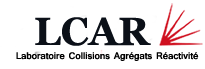# Laboratoire Collisions Agrégats Réactivité - UMR5589

Université Paul Sabatier - Bat. 3R4 - 118 route de Narbonne 31062 Toulouse Cedex 09, France

## Index of refraction of gases for matter waves : Effect of the motion of the gas particles on the calculation of the index

Two different formulas relating the index of refraction n of gases for atom waves to the scattering amplitude have been published. We show here that these two formulas are not consistent with the definition of the total scattering cross section while the formula developed by one of us [C. Champenois, Ph.D. thesis, Université P. Sabatier, 1999 (unpublished)] is in agreement with this standard knowledge. We discuss this result, in particular in the neutron case for which such an index was first introduced. We finally evaluate the index of refraction as a function of well-known quantities and we discuss the order of magnitude of the ratio of (n−1)/nt, where nt is the gas density.

http://hal.archives-ouvertes.fr/hal...

Voir en ligne : Phys. Rev. A 77, 013621 (2008)